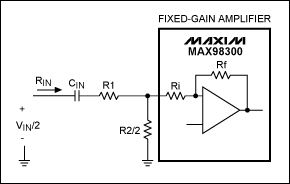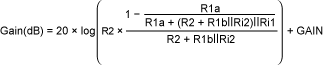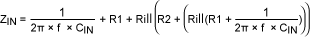# Resistor Network Sets Gain for Fixed-Gain Differential Amplifier

### Abstract

By adding an external resistor network you can lower the gain of a fixed-gain differential amplifier (like the MAX98300) to the gain level required, but you must account for the loading effects of internal resistors. This note includes equations for calculating these effects, plus a link to a spreadsheet for selecting resistor values needed in the network.

A similar design idea appeared in the July 20, 2009, issue of EDN magazine.

When a fixed-gain amplifier does not offer the optimum gain for an application, you can adjust the gain to a lower value by adding an external resistor network. This attenuation circuit works like a voltage-divider, but with a key difference: the external network is loaded down by input resistors internal to the fixed-gain amplifier (Figure 1). For the differential-input configuration, this system can be reduced to an equivalent half circuit for analysis (Figure 2).Voltage gain for the differential configuration (Figure 1A) is determined as follows:Where GAIN is the amplifier's fixed voltage gain in dB. (The MAX98300 differential audio amplifier is available in fixed-gain versions of 0, 3, 6, 9, and 12dB; its input resistance is typically 20kΩ in 6, 9, and 12dB configurations, 28kO in 3dB configuration, and 40kΩ in 0dB configuration.)

For single-ended configurations, the gain isThese gain equations assume that the frequency is much higher than the cutoff frequency of the highpass filter formed by CIN and the equivalent input resistance of the circuit.

The fixed gain of the MAX98300 amplifier is typically within 5%; the internal input resistors have an absolute tolerance of ±50%, which must be considered when calculating the resulting system gain. The tolerance of external resistors must also be accounted for by using a worst-case approach for calculating the gain tolerance. Replacing the resistor values with their maximum deviations from normal (i.e., the extreme values at the ± tolerance limits) yields the worst-case scenario:

 Lowest Gain Single-Ended Differential Highest Gain Single-Ended Differential R1a +Tolerance +Tolerance R1a -Tolerance -Tolerance R1b +Tolerance +Tolerance R1b -Tolerance -Tolerance R2 -Tolerance -Tolerance R2 +Tolerance +Tolerance Ri1 -Tolerance -Tolerance Ri1 +Tolerance +Tolerance Ri2 +Tolerance -Tolerance Ri2 -Tolerance +Tolerance

For the tightest tolerance, choose a small R1 with respect to Ri. Make sure to account for the source characteristics of VIN, which must be able to drive the equivalent load of the network. When determining final gain for the system, remember that the output impedance of VIN forms a voltage-divider with the input impedance of the attenuation circuit. This load (RIN) can be determined as follows.

For the differential configuration, RIN = R1 + Ri/(R2/2). RIN and CIN form a highpass filter whose cutoff frequency, F-3dB, is: F-3dB = 1/(2 × π × RIN × CIN).

For the single-ended configuration, RIN = R1 + Ri/(R2 + R1/Ri). Input impedance (ZIN) for the attenuation network is:Where f is the input-signal frequency.

Deriving the highpass cutoff frequency for the single-ended case is less straightforward. To find F-3dB you must know the resistor values. Once they are known, you can solve the following equation for f = F-3dB: ZIN(f) = √2 × ZIN(f = 5000).

A spreadsheet is useful for determining the correct resistor values and system tolerances. Remember that resistors come in discrete values of resistance. They must be matched rather than simply calculated. A spreadsheet for determining custom gains and gain tolerances for both single-ended and differential configurations of the MAX98300 is available for download.